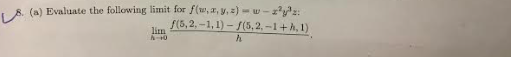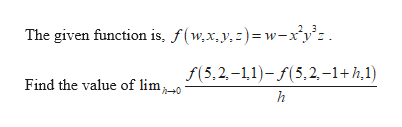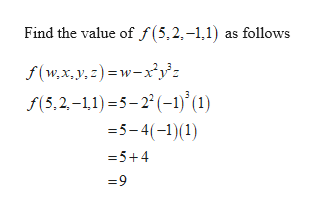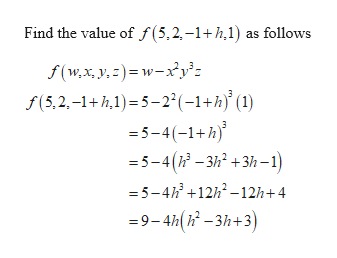# | 8. (a) Evaluate the following limit for f(w, a, y, 2) - w-zy:f(5,2.-1,1) - S(5,2, -1+A, 1)lim

Question
1 viewshelp_outlineImage Transcriptionclose| 8. (a) Evaluate the following limit for f(w, a, y, 2) - w-zy: f(5,2.-1,1) - S(5,2, -1+A, 1) lim fullscreen
check_circle

Step 1help_outlineImage TranscriptioncloseThe given function is, f(w,x,y,z)= w-x°y°z. f(5,2,-11)- f(5,2, –1+h,1) Find the value of limo fullscreen
Step 2help_outlineImage TranscriptioncloseFind the value of f (5,2,-1,1) as follows f(w,x,y,z) =w-x²y}: f(5,2,-11) =5-2° (-1)’(1) =5-4(-1)(1) =5+4 =9 fullscreen
Step 3help_outlineImage TranscriptioncloseFind the value of f(5,2,-1+h,1) as follows f(w,x, y, z) = w-xy': f(5,2,–1+h,1)= 5–2°(-1+h) (1) = 5-4(-1+h) = 5-4(h - 3h? +3h 1) = 5-4h +12h? -12h+4 =9-4h(k – 3h+3) fullscreen

### Want to see the full answer?

See Solution

#### Want to see this answer and more?

Solutions are written by subject experts who are available 24/7. Questions are typically answered within 1 hour.*

See Solution
*Response times may vary by subject and question.
Tagged in